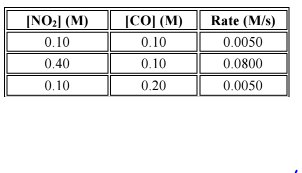# Problem: Many gaseous reactions occur in a car engine and exhaust system. One of these is represented by the following equation: NO2 (g) + CO (g) ⇄ NO (g) + CO2 (g) What is the rate of the reaction if the concentrations of NO2 and CO are 0.25 M and 0.30 M respectively?  For the choices below, what is the order of reaction for NO2?

###### FREE Expert Solution
82% (187 ratings)
###### Problem Details

Many gaseous reactions occur in a car engine and exhaust system. One of these is represented by the following equation:

NO2 (g) + CO (g) ⇄ NO (g) + CO2 (g)

What is the rate of the reaction if the concentrations of NO2 and CO are 0.25 M and 0.30 M respectively?

For the choices below, what is the order of reaction for NO2?What scientific concept do you need to know in order to solve this problem?

Our tutors have indicated that to solve this problem you will need to apply the Rate Law concept. You can view video lessons to learn Rate Law. Or if you need more Rate Law practice, you can also practice Rate Law practice problems.

What is the difficulty of this problem?

Our tutors rated the difficulty ofMany gaseous reactions occur in a car engine and exhaust sys...as high difficulty.

How long does this problem take to solve?

Our expert Chemistry tutor, Dasha took 10 minutes and 15 seconds to solve this problem. You can follow their steps in the video explanation above.

What professor is this problem relevant for?

Based on our data, we think this problem is relevant for Professor Dixon's class at UCF.